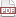### Tip 54: Put functions in your structs

17 January 12. [link]PDF version

level: your structures are getting diverse
purpose: maintain a standard form over diversity

Part of a series of tips on POSIX and C. Start from the tip intro page, or get 21st Century C, the book based on this series.

Since the last two episodes included some long sample code, this time I'm going to just tell you about Apophenia, the library of stats functions that co-evolved with Modeling with Data.

One of the key data structures is intended to represent a statistical model. Broadly, statistical models are all the same: the box diagram would have parameters on one side (think of the mean and variance of a Normal distribution, μ and σ), then another arrow for the data (a data point, x), and the black box would spit out a probability, P(x| μ, σ).

So our struct will have elements named parameters and data, which are not especially challenging. There should be a probability function to go from parameters and data to P(parameters, data), and here we run into a problem: there is a different calculation for every model. The math for a Normal distribution has nothing to do with the math for a Poisson distribution or a Binomial distribution. So having a single function prob(parameters, data, model) won't work.

The cleanest solution is to put a slot for the function inside the struct typedef, and associate a new function with every struct:

typedef double (*model_to_double)(apop_model *);

typedef struct {
apop_data *parameters, *data;
model_to_double *prob, *log_likelihood;
} apop_model;


Simulation models typically use the probability, but people who work with probability distribution-based models tend to work with the log probability (i.e., the log likelihood, where the distinction between probability and likelihood is irrelevant for our purposes), so I threw in a slot for both.

Now, when we define a new model object, we set the functions as needed.

/* This is very cut down from the Apophenia source, and I'm not going
to explain the math here.
Focus on the declaration below the function.
*/
static double apply_me(double x, void *mu){ return gsl_pow_2(x - *(double *)mu); }

static double normal_log_likelihood(apop_data *d, apop_model *params){
//check that params->parameters is not null here.
double mu = apop_data_get(params->parameters, 0, -1);
double sd = apop_data_get(params->parameters, 1, -1);
return -apop_map_sum(d, .fn_dp = apply_me, .param = &mu)/(2*gsl_pow_2(sd))
- tsize*(M_LNPI+M_LN2+log(sd));
}

apop_model apop_normal= {.name="Normal distribution", .log_likelihood=normal_log_likelihood};


Hey, wait--after all that I didn't define the probability function. But it's easy to calculate: the log probability is log(probability), and probability = exp(log likelihood). Instead, here is a dispatch function prob, which lives outside of the struct, and would be used the way all the functions worked before we started putting methods inside structs. We usually see the struct as the first input to such functions, but Apophenia's rule for ordering the inputs is that the data always comes first.

double prob(apop_data *d, apop_model *p){
assert(p->parameters); //I expect users have set this before calling.
if (p->prob)
return p->prob(d, p);
else if (p->log_likelihood)
return exp(p->log_likelihood(d, p));
else Apop_error("I need either the prob or log likelihood "
"methods in the input model.");
}


If there were a sensible default method for calculating the probability given data and parameters, we'd put it here in the dispatch function. For the estimation of a model (find the most likely parameters given data), there actually is a default, in the form of maximum likelihood methods. So the estimate routine looks like

//again, this is cut.
apop_model * apop_estimate(apop_data *d, apop_model *p){
if (p->estimate)
return p->estimate(d, p);
else
return apop_maximum_likelihood(d, p);
}


If we put a function inside of the struct, then we need to point the struct to the right function on initialization every time. If we can reasonably expect that the function will be different every time, then that's exactly what we need.

If you have a new_struct function that gathers together the input data and functions and spits out a cleaned-up struct, then you can use that setup function to assign a default function, the way that apop_estimate used the maximum likelihood function when the struct didn't have its own estimate routine.

I prefer to set up structures using designated initializers, so that means I need a dispatch function that checks for the method being called and uses a default as needed.

###### I want this
Further, let me point out a sad fact about methods inside of structs. You pretty much always need to send in the struct itself, which means redundancy. If we didn't have the dispatch function, we'd have redundant calls like normal_dist.p(data, normal_dist).

C++-type languages have a special rule that the first element of a function inside a struct will be the struct itself. Either you write a method with the appropriate first argument, and so a header like normal_estimate(apop_model this, apop_data *d); or the system may just define a special variable this to point to the parent struct.

C doesn't define magic variables for you, and it is always honest and transparent about what parameters get sent in to a function. Normally, if we want to futz around with the parameters of a function, we do it with the preprocessor, which will gladly rewrite f(anything) to f(anything else). However, all of the transformations are a function of what goes on inside of the parens. There's no way to get the preprocessor to transform the text s.prob(d) to s.prob(s, d). If you don't want to slavishly imitate C++-type syntax, you can write a macro

#define prob(s, ...) s.prob(s, __VA_ARGS__)


But now you've cluttered up the global namespace with this prob symbol. So there's one more reason to use a dispatch function: it can take care of the redundancy for you.